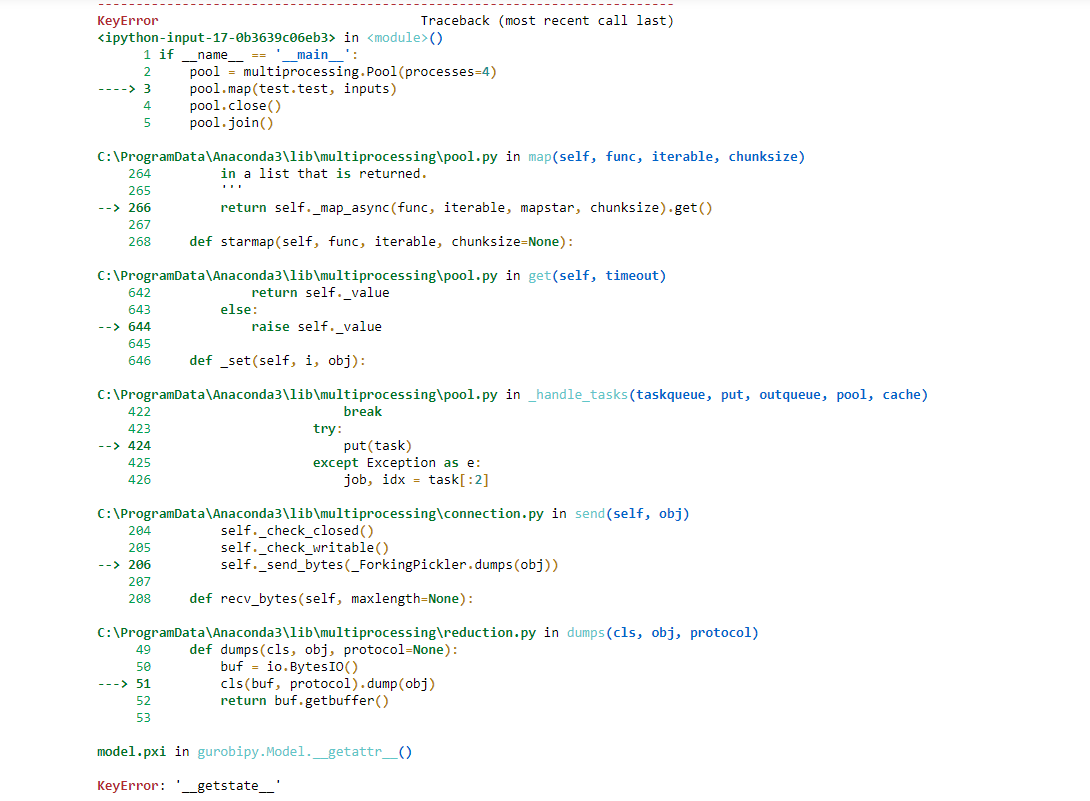•Hi Boyu,

Before trying to run in parallel, I recommend testing your models one after the other. They are very small and even sequentially, Gurobi should solve all 48 of them in just a few seconds.

Furthermore, you need to enable the shared memory option in joblib to make it possible to write to the dictionaries you defined for the solution output:

Parallel(n_jobs=num_cores, require='sharedmem')

You also need to check the solving status before querying the objective value:

if m[i].Status == GRB.OPTIMAL:
object_val[i]=m[i].objVal
else:
object_val[i]=''

In summary, I cannot recommend running Gurobi in parallel like this. For harder problems, single jobs will fight for computing resources and you might run into all kinds of hard to debug situations. You should get better performance by just solving the models sequentially.

Cheers,
Matthias
•Hi Matthias,

Currently, I am trying another way to do that but "KeyError: '__getstate__'"  happened.

First, I start building 5 LPs sequentially:

from gurobipy import *
from gurobipy import GRB

a={1:2,2:2,3:8,4:7,5:3}
b={1:3,2:5,3:6,4:8,5:5}
c={1:4,2:2,3:3,4:5,5:7}
d={1:1,2:7,3:3,4:2,5:9}
object_val={}
x={}
y={}
z={}
m={}

for i in [1,2,3,4,5]:
# Create a new model
m[i]=Model()

# Create variables

# Set objective
m[i].setObjective(x[i] + y[i] + 2 * z[i] , GRB.MAXIMIZE)

# Add constraint: x + a y + b z <= c
m[i].addConstr(x[i] + a[i] * y[i] + b[i] * z[i] <= c[i])

# Add constraint: x + y >= 1

Second, I defined the function to solve a single LP model and save it as "test.py":

def test(i):

# Optimize model
m=i
m.optimize()
return m.objVal

Third, I create the input data for the function will solved by parallel:

inputs=[]
for i in [1,2,3,4,5]:
inputs.append([i,m[i]])

Finally, I tried to use "multiprocessing" package to solve these 5 LPs parallelly:

import test
import multiprocessing
if __name__ == '__main__':
pool = multiprocessing.Pool(processes=4)
pool.map(test.test, inputs)
pool.close()
pool.join()
print('done')

However, an error occurs, it said "KeyError: '__getstate__'"